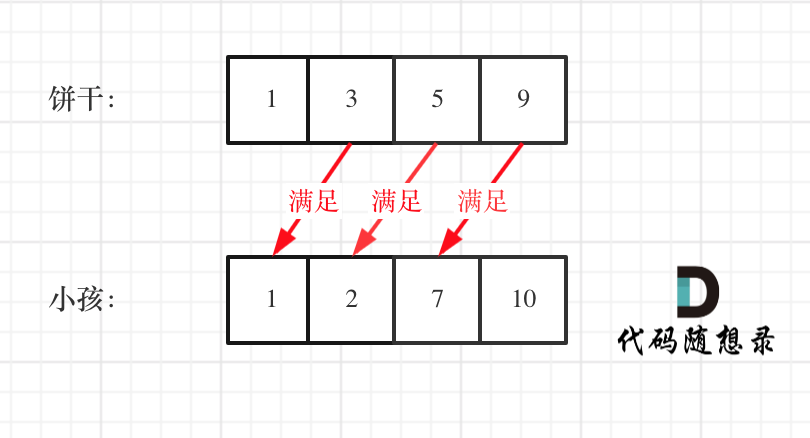## # 455.分发饼干

• 1 <= g.length <= 3 * 10^4
• 0 <= s.length <= 3 * 10^4
• 1 <= g[i], s[j] <= 2^31 - 1

## # 思路C++代码整体如下：

``````// 时间复杂度：O(nlogn)
// 空间复杂度：O(1)
class Solution {
public:
int findContentChildren(vector<int>& g, vector<int>& s) {
sort(g.begin(), g.end());
sort(s.begin(), s.end());
int index = s.size() - 1; // 饼干数组的下表
int result = 0;
for (int i = g.size() - 1; i >= 0; i--) {
if (index >= 0 && s[index] >= g[i]) {
result++;
index--;
}
}
return result;
}
};
``````
1
2
3
4
5
6
7
8
9
10
11
12
13
14
15
16
17
18

``````class Solution {
public:
int findContentChildren(vector<int>& g, vector<int>& s) {
sort(g.begin(),g.end());
sort(s.begin(),s.end());
int index = 0;
for(int i = 0;i < s.size();++i){
if(index < g.size() && g[index] <= s[i]){
index++;
}
}
return index;
}
};
``````
1
2
3
4
5
6
7
8
9
10
11
12
13
14

## # 其他语言版本

Java：

``````class Solution {
// 思路1：优先考虑饼干，小饼干先喂饱小胃口
public int findContentChildren(int[] g, int[] s) {
Arrays.sort(g);
Arrays.sort(s);
int start = 0;
int count = 0;
for (int i = 0; i < s.length && start < g.length; i++) {
if (s[i] >= g[start]) {
start++;
count++;
}
}
return count;
}
}
``````
1
2
3
4
5
6
7
8
9
10
11
12
13
14
15
16
``````class Solution {
// 思路2：优先考虑胃口，先喂饱大胃口
public int findContentChildren(int[] g, int[] s) {
Arrays.sort(g);
Arrays.sort(s);
int count = 0;
int start = s.length - 1;
// 遍历胃口
for (int index = g.length - 1; index >= 0; index--) {
if(start >= 0 && g[index] <= s[start]) {
start--;
count++;
}
}
return count;
}
}
``````
1
2
3
4
5
6
7
8
9
10
11
12
13
14
15
16
17

Python：

``````class Solution:
# 思路1：优先考虑胃饼干
def findContentChildren(self, g: List[int], s: List[int]) -> int:
g.sort()
s.sort()
res = 0
for i in range(len(s)):
if res <len(g) and s[i] >= g[res]:  #小饼干先喂饱小胃口
res += 1
return res
``````
1
2
3
4
5
6
7
8
9
10
``````class Solution:
# 思路2：优先考虑胃口
def findContentChildren(self, g: List[int], s: List[int]) -> int:
g.sort()
s.sort()
start, count = len(s) - 1, 0
for index in range(len(g) - 1, -1, -1): # 先喂饱大胃口
if start >= 0 and g[index] <= s[start]:
start -= 1
count += 1
return count
``````
1
2
3
4
5
6
7
8
9
10
11

Go：

``````//排序后，局部最优
func findContentChildren(g []int, s []int) int {
sort.Ints(g)
sort.Ints(s)

// 从小到大
child := 0
for sIdx := 0; child < len(g) && sIdx < len(s); sIdx++ {
if s[sIdx] >= g[child] {//如果饼干的大小大于或等于孩子的为空则给与，否则不给予，继续寻找选一个饼干是否符合
child++
}
}

return child
}
``````
1
2
3
4
5
6
7
8
9
10
11
12
13
14
15

Javascript:

``````var findContentChildren = function(g, s) {
g = g.sort((a, b) => a - b)
s = s.sort((a, b) => a - b)
let result = 0
let index = s.length - 1
for(let i = g.length - 1; i >= 0; i--) {
if(index >= 0 && s[index] >= g[i]) {
result++
index--
}
}
return result
};

``````
1
2
3
4
5
6
7
8
9
10
11
12
13
14